Get instant live expert help with Excel or Google Sheets“My Excelchat expert helped me in less than 20 minutes, saving me what would have been 5 hours of work!”

#### Post your problem and you’ll get expert help in seconds.

Your message must be at least 40 characters
Our professional experts are available now. Your privacy is guaranteed.

# How to Sort Numbers in Ascending or Descending Order in Excel

There are times when you need to sort your large data in an ascending or descending order. By sorting your data in ascending or descending order, you can quickly find information on your list. You can use the “expanding range” to get this function done effectively.

## How to sort numbers in ascending or descending order

To sort data in Excel numerically, text in alphabetical order, sort range in ascending or descending order, the “SMALL”, “LARGE” with an “expanding range” function can be used.

Sorting your data is vital in:

• Payroll processing
• Preparation of financial documents

### How to Sort Data with the Small Function in Ascending Order

The “SMALL” Function with an “expanding range” is especially crucial for picking out the smallest value in your list of data.

However, a simple sort can do this for you, but the expanding range is excellent because it accommodates any changes you make in your data any time in the future and automatically changes the order to suit this change.

Basic formula` =``SMALL(data,ROWS (exp_rng))`

• Example 1: Using the SMALL function to sort C6:C13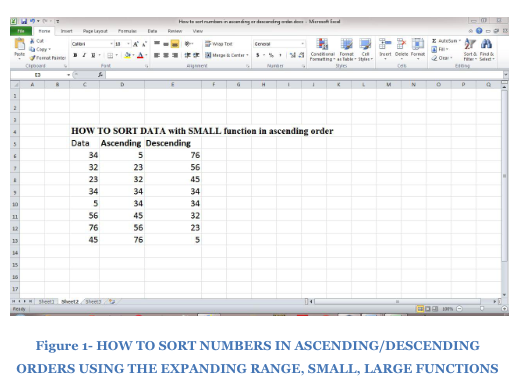Figure 1 shows a given set of data sorted in ascending and descending order; we used a basic formula by incorporating the SMALL function using the expanding range in a simplified process:

`= SMALL(C6:C13,ROWS(\$C\$6:C6))`

### Step 1

• Now place your cursor at the adjacent cell, or like in the figure below on Cell D6. Click on fx as shown in figure 2 below.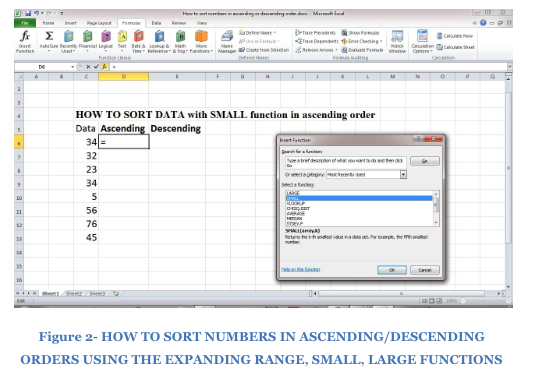• Select SMALL in the insert function pop up
• Click on the “OK” as shown in figure 2
• You will see this figure below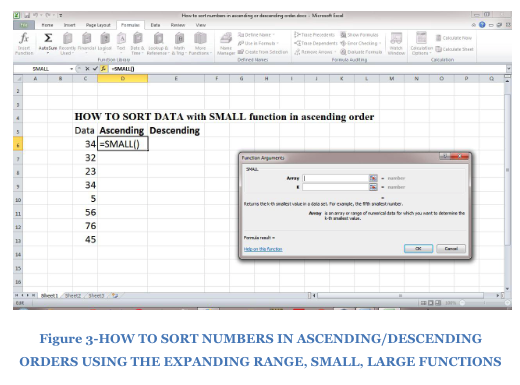### Step 2

• Type in the Array as shown; \$C6:\$C13,
• Next, type in the “K” number; this should be several figures below your estimated small value
• If you pick your smallest value, then your data will be entered without selecting for your smallest value. Look at the figure below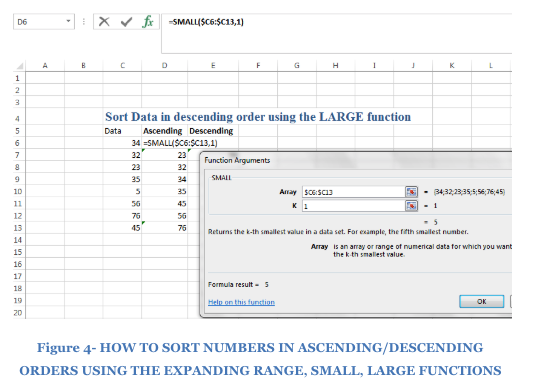• Click OK
• Your smallest figure is displayed as seen below.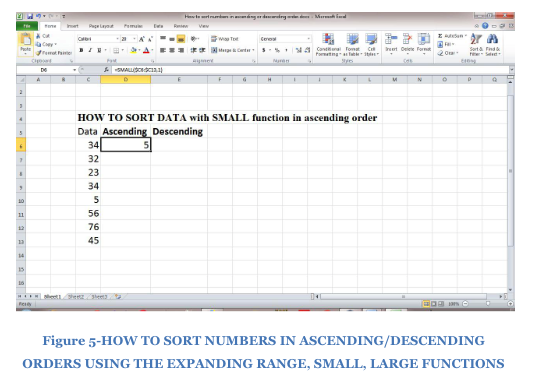### Step 3

• To further fill the next cell below, simply copy the text
• Now paste it in the next cell as shown below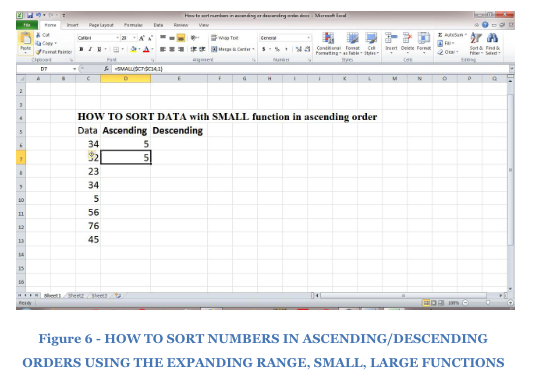• Now, take your cursor and place in the fx section, That is the =SMALL (\$C7:\$C14,1)
• Change the k value from 1 to 2 as shown below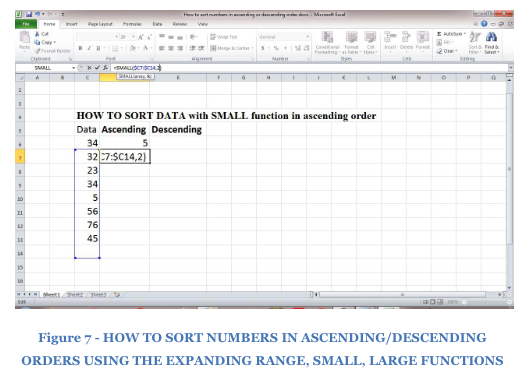• Press the Enter key and this should select your second value in ascending order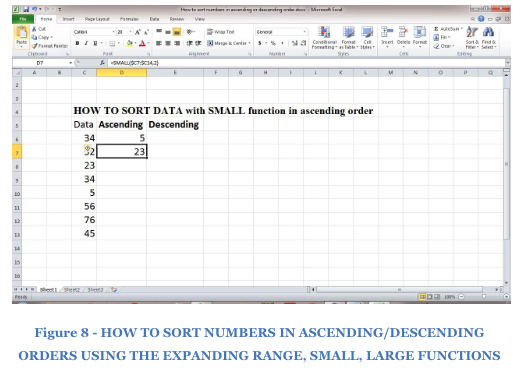### Step 4

• To pick the next cells, simply copy the text again and paste in the next section, changing the K from 2 to  3 and so on
• Your formulas for each cell should look like this or you can manually use the formulas below:

`=SMALL (\$C7:\$C14,1)`

`=SMALL (\$C7:\$C14,2)`

`=SMALL (\$C7:\$C14,3)`

`=SMALL (\$C7:\$C14,4)`

As you copy the SMALL function formula down the table, the table expands in terms of the incrementing value.

## Sort Data in descending order using the LARGE function

You can equally sort numbers in descending order as shown in figure 1. All you need do is replace the SMALL function with the LARGE function:

Basic formula:` =``LARGE(data,ROWS( exp_rng))`

### Example 2- Using the LARGE function to sort data in descending order

The LARGE function for figure 1 will be thus:

`=LARGE(C6:C13,ROWS (\$C\$6:C\$))`

Step 1

• Again, enter your data as shown in figure 1
• Next, place your cursor on Cell E6 or as shown and click the fx function simultaneously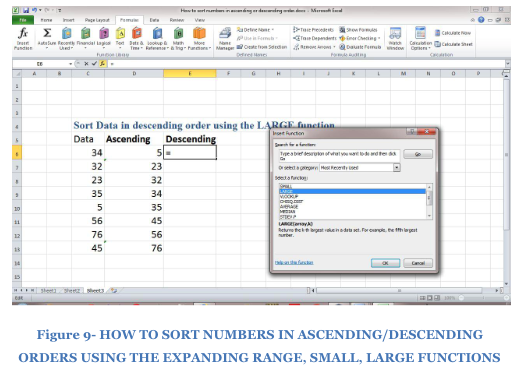• Select the LARGE function shown above
• Enter the Array value; \$C6:\$C13
• Enter the K value, again this should be 1, so the highest value will be selected. All of this is shown below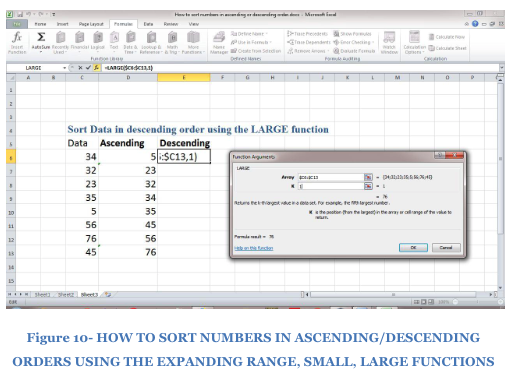• Click OK to enter the function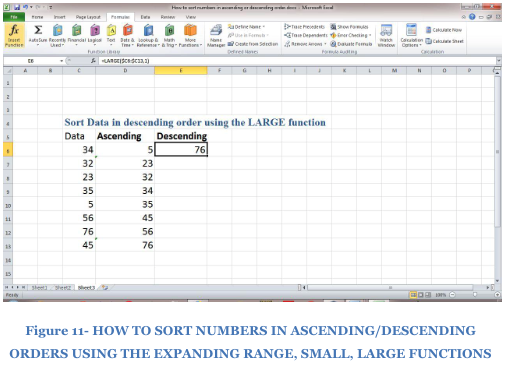### Step 2

• In the same way as the SMALL function, copy the Cell E6
• Paste in Cell E7 as shown in the figure below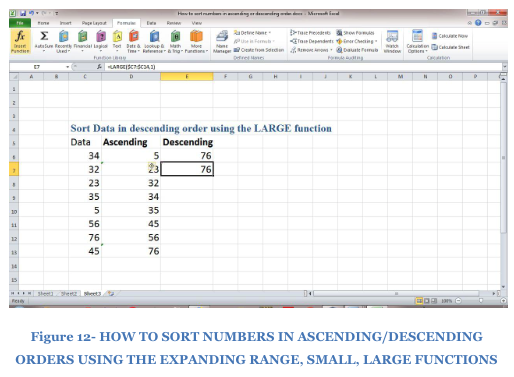• Highlight the K value and change it to 2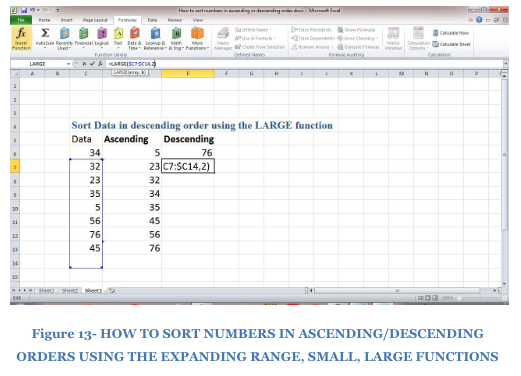• Next, click OK to enter to formula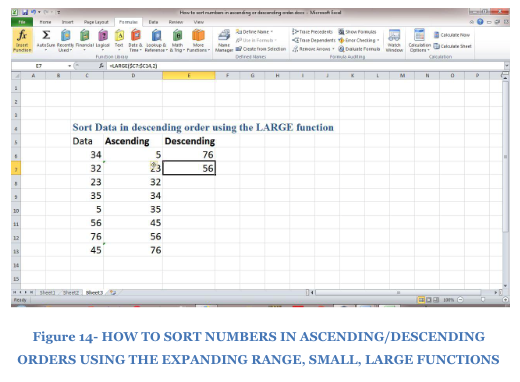### Step 3

1. To select the next values in decreasing order, copy the LARGE function from the cell to the next blank cell
2. Highlight and change your figures as you move further

It should look like this:

`=LARGE(\$C7:\$C14,1)`

`=LARGE(\$C7:\$C14,2)`

`=LARGE(\$C7:\$C14,3)`

`=LARGE(\$C7:\$C14,4)`

Like the SMALL function, the LARGE function extracts your “nth” value and arranges your values in “nth largest” or descending order.

Still need some help with Excel formatting or have other questions about Excel? Connect with a live Excel expert here for some 1 on 1 help. Your first session is always free.

### Did this post not answer your question? Get a solution from connecting with the expert.Another blog reader asked this question today on Excelchat:
Related blogs
Solution examplesI need to create a pivot table that sums visits per day by provider: Date...Visits....Provider So, I can ask...how many visits did provider X have on day Y?
Solved by F. J. in 34 minscould someone breakdown this formula for me :D =ArrayFormula(IF(D\$5>=ROWS(B\$5:B5),INDEX(Sheet1!C\$6:C\$21,SMALL(IF(Sheet1!D\$6:D\$21=\$E\$5,ROW(Sheet1!C\$6:C\$21)-ROW(Sheet1!C\$6)+1),ROWS(B\$5:B5))),""))
Solved by C. C. in 40 minsI use a Google Sheet to keep track of prospective investors. The first tab functions as a master tab. Some of the columns I use to categorize the prospects. For example, if they are a candidate to lead for the next round, in the column, I would put a y for Yes. Then, I would like to have a separate tab that shows only those investors that I have flagged as possible lead investors.
Solved by I. H. in 40 minsI need to create a rows in Sheet 1 where Sheet 2 column c has values in Sheet 2 column D that are greater than .01 and leave out items in Sheet 1 where Sheet 3 column D are = 0 (without using a filter, then copy/paste).
Solved by I. Y. in 21 minsI need a formula that looks for a specific name in three columns, takes all of the rows that match to get dates from another column. Then from the dates that match the rows with the names, take the second earliest date and generate it. The below formula is giving me the second date listed for the specific person instead of giving me the second earliest date. =IFERROR(INDEX('Input Data'!\$E\$5:\$E\$150,SMALL(IF(Tracker!\$B6='Input Data'!\$G\$5:\$I\$150,ROW('Input Data'!\$G\$5:\$I\$150)-ROW('Input Data'!\$G\$5)+1),2)), "N/A")
Solved by A. J. in 34 mins## Subscribe to Excelchat.coAnother blog reader asked this question today on Excelchat: